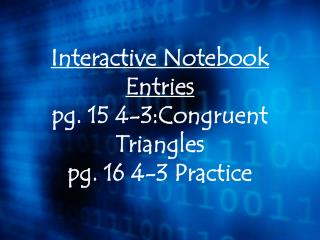Download PresentationInteractive Notebook Entries pg. 15 4-3:Congruent Triangles pg. 16 4-3 Practice

# Interactive Notebook Entries pg. 15 4-3:Congruent Triangles pg. 16 4-3 Practice

Download Presentation## Interactive Notebook Entries pg. 15 4-3:Congruent Triangles pg. 16 4-3 Practice

- - - - - - - - - - - - - - - - - - - - - - - - - - - E N D - - - - - - - - - - - - - - - - - - - - - - - - - - -
##### Presentation Transcript

1. Interactive Notebook Entries pg. 15 4-3:Congruent Trianglespg. 16 4-3 Practice

2. 4.3 Congruent Triangles Objectives: …name and label corresponding parts of congruent triangles. …identify congruence transformations.

3. Corresponding Parts of Congruent Triangles are Congruent (CPCTC) Two triangles are congruent if and only if their corresponding parts are congruent. Triangles that are the same size and shape are congruent triangles.

4. B Y Z C X A Corresponding parts of congruent triangles Name all the corresponding parts of ABC and XYZ.

5. B E C F A D IDENTIFY CONGRUENCE TRANSFORMATIONS If you slide ΔABC down and to the right, it is still congruent to ΔDEF. B C A

6. B E C F A D IDENTIFY CONGRUENCE TRANSFORMATIONS If you turn ΔABC, it is still congruent to ΔDEF. A B C

7. B E C F A D IDENTIFY CONGRUENCE TRANSFORMATIONS If you flip ΔABC, it is still congruent to ΔDEF. A C B

8. COORDINATE GEOMETRYThe vertices of RSTare R(─3, 0), S(0, 5), and T(1, 1). The vertices of RSTare R(3, 0), S(0, ─5), and T(─1, ─1). Verify that RST RST. Example 3-2a

9. Example 3-2b Use the Distance Formula to find the length of each side of the triangles.

10. Example 3-2b Use the Distance Formula to find the length of each side of the triangles.

11. Example 3-2b Use the Distance Formula to find the length of each side of the triangles.

12. a. Verify that ABC ABC. Example 3-2f COORDINATE GEOMETRYThe vertices of ABC are A(–5, 5), B(0, 3), and C(–4, 1). The vertices of ABCare A(5, –5), B(0, –3), and C(4, –1). Answer:

13. Example 3-2g b.Name the congruence transformation for ABC and ABC. Answer:turn